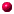This is the official website of The INTERNATIONAL LEAGUE OF ATOMIC WOMENRadioactivity is the spontaneous transformation of an unstable atom and often results in the emission of radiation. This process is referred to as a transformation, a decay or a disintegration of an atom.Absorbed dose is a measure of the amount of energy from an ionizing radiation deposited in a mass of some material. Dose is affected by the type and the amount of radiation as well as by the physical properties of the material itself. Normally measured in units of Gray (RAD).Effective and equivalent doses, allow the comparison of different tissues or types of radiation. Normally measured in units of Sievert (Rem).Common U.S. units: These are the common units used in the United States in health physics.Roentgen (R): Is a unit for measuring the amount of gamma or X rays in air. It is a measure of the ionizations of the molecules in a mass of air. Describes a quantity called exposure. The main advantage of this unit is that it is easy to measure directly, but it is limited because it is only for deposition in air, and only for gamma and x rays.Rad (radiation absorbed dose), is defined as the absorption of 100 ergs per gram of material. One RAD measures a quantity called absorbed dose of radiation, and relates to the amount of energy actually absorbed per gram of tissue, which is equal to about 83% of the Roentgen value. It can be used for any type of radiation or material. The unit does not describe the biological effects of different form of radiation. A millirad or mrad is a thousandth of a rad.REM (Roentgen Equivalent Man), is a unit used to derive a quantity called "equivalent dose" and compare the effective biological damage of the radiation dose produced by different particles. This relates the absorbed dose in human tissue. Not all radiation has the same biological effect, even for the same amount of absorbed dose. Often expressed in millirem (a thousandth of a rem).Equivalent dose (rem), is the result of multiplying absorbed dose (RAD) by a quality factor (Q) that is unique to the of incident radiation.

Q: a factor reflecting our notion of how harmful the particular type of radiation. A given dose of radiation with a higher Q is more damaging than the same dose with a smaller Q.

The relation between rad and rem depends on the kind of particle emitting the radiation:
for gamma rays, 1 rad = 1 rem for beta, 1 rad = 10 rem for alpha, 1 rad = 30 remCurie (Ci), is a unit used to measure a radioactivity. One curie is the amount of radioactivity in one gram of Radium, the element first discovered by Madame Curie. It is the number of disintegrations taking place each second in 1 gram of radium, leading to the emission of 37.000.000.000 gamma rays or other particles every second.

It is also the quantity of a radioactive material that will have 37.000.000.000 transformations -or Becquerels- in one second. One Becquerel is equal to one disintegration per second. There are 37.000.000.000 Becquerels in one curie.

Often radioactivity is expressed in smaller units like:
Millicurie (mCi): one thousandth of a Curie or.
Microcurie (uCi): one millionth of a Curie or
Picocurie (nCi): one billionth of of a curie.

Radiation is often measured in one of these three units, depending on what is being measured and why.In S.I., international standard units: These would be Coulombs/kg for roentgen, Grays for rads and Sieverts for rem. These are the common units used throughout the world in health physics.Gray (Gy): measures the absorbed dose. One gray is equivalent to 100 rads; one rad = 0.01 gray, also called "one centi-gray". It is equal to one joule of energy deposited in one kg of a material.Sievert (Sv): used to derive equivalent dose. One sievert = 100 rem

Equivalent dose in Sv = absorbed dose in Gy x (Q), where (Q) is a quality factor that is unique to the of incident radiation.

SI Prefixes: Many units are broken down into smaller units or expressed as multiples, using standard metric prefixes.

Home

 DISCLAIMER Atomic Women is designed for educational purposes only and is not engaged in rendering medical advice. The information provided through this web site should not be used for diagnosing or treating a disease. It is not a substitute for professional care. If you have or suspect you may have a health problem, you should consult your doctor.